# Bicycle wheel

After driving 157 m bicycle wheel rotates 100 times. What is the radius of the wheel in cm?

r =  25 cm

### Step-by-step explanation: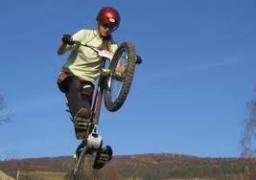Did you find an error or inaccuracy? Feel free to write us. Thank you!#### You need to know the following knowledge to solve this word math problem:

We encourage you to watch this tutorial video on this math problem:

## Related math problems and questions:

• Bicycle wheel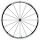Bicycle wheel diameter is 62 cm. How many times turns the bicycle on the road 1 km long?
• Bike wheel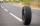The bike wheel has a radius of 30cm. How many times does it turn if we go on a 471m bike?
• A bicycle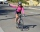A bicycle wheel has a diameter of 63 cm. Calculate how many times the wheel turns round in travelling 19.8 km .
• Ferris wheel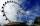Ferris wheel reaches to 22 m tall and moves at the speed of 0.5m/s. During one drive wheel rotates three times. What is the total drive time?
• Bicycle wheels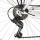The driving wheel of a bicycle has 54 teeth. The driven wheel has 22 teeth. After how many revolutions will meet the same teeth?Calculate the radius of the circle whose length is 107 cm larger than its diameter
• Velocipede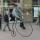The front wheel of velocipede from the year 1880 had a diameter 1.8 m. Suppose the front wheel turned again one, then the rear wheel 6 times. What was the diameter of the rear wheel?
• Wheel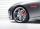How many times turns the wheel of a passenger car in one second if car run at speed 100 km/h. Wheel diameter is d = 62 cm.
• Wheel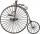Diameter of motocycle wheel is 52 cm. How many times rotates wheel on roand long 2 km?
• Animal species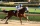Of 100 types of animals, 9/100 were discovered in ancient times, and 2/100 were discovered in the Middle Ages. Another 3/10 were discovered in the 1800s. What fraction of the 100 types of animals was discovered after the 1800s? Explain.
• Height of the cylinderThe cylinder volume is 150 dm cubic, the base diameter is 100 cm. What is the height of the cylinder?
• Wheel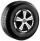What is the wheel diameter if on the 0.57 km track turns 106 times?
• Bicycle gears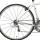The toothed wheel on the bicycle pedal has 40 teeth, the wheel on the rear wheel has only 16 teeth. How many times does the rear wheel turn if the pedals rotate 50 times?
• GearsThe front gear on the bike has 32 teeth and the rear, on the wheel, has 12 teeth. How many times does the bike's rear-wheel turn if you turn the right pedal 30 times? What distance will you go if the circumference of the bicycle wheel is 250 cm?
• ThalesThales is 1 m from the hole. The eyes are 150 cm above the ground and look into the hole with a diameter of 120 cm as shown. Calculate the depth of the hole.
• TableclothsThe restaurant has sixty-two square tablecloths with a side length of 150 cm and 36 rectangular tablecloths with dimensions of 140 cm and 160 cm. A) How many meters of hemming ribbon will be needed if we add 50 cm to each tablecloth? B) The ribbon sale in
• Athlete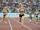How long length run athlete when the track is circular shape of radius 120 meters and an athlete runs five times in the circuit?